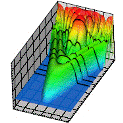## Research Papers in Physics and Astronomy## Anthony F. Starace Publications

January 1980

#### Comments

Published in Physica Scripta 21 (1980), pp. 368–372. Copyright © 1980 Royal Swedish Academy of Science; published by Institute of Physics Publishing. Used by permission. http://www.iop.org/EJ/journal/PhysScr

#### Abstract

A graphical procedure is presented for calculating first order transition matrices for a general (open-shell) atom. The first order transition matrix may be used to calculate matrix elements of a general one-body operator of rank λ in orbital space and σ in spin space. In the random phase approximation we obtain a set of N + N′ coupled differential equations for N final state radial functions and N′ initial state radial functions which completely determine the first order transition matrix for an atomic system having N final state channels. (The relation of N′ to N is dependent on the atomic system studied.) These N + N′ differential equations reduce to familiar forms in the following cases: (1) When initial state correlations are ignored, we obtain the N coupled differential equations of the Close-Coupling Approximation; (2) When the atom has only closed subshells we obtain N′ = N and the 2N coupled differential equations are those obtained in the Chang-Fano version of the Random Phase Approximation.

COinS